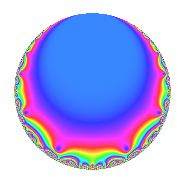# Properties

 Label 1003.2.a.bLevel 1003 Weight 2 Character orbit 1003.a Self dual Yes Analytic conductor 8.009 Analytic rank 1 Dimension 1 CM No Inner twists 1

# Learn more about

## Newspace parameters

 Level: $$N$$ = $$1003 = 17 \cdot 59$$ Weight: $$k$$ = $$2$$ Character orbit: $$[\chi]$$ = 1003.a (trivial)

## Newform invariants

 Self dual: Yes Analytic conductor: $$8.00899532273$$ Analytic rank: $$1$$ Dimension: $$1$$ Coefficient field: $$\mathbb{Q}$$ Coefficient ring: $$\mathbb{Z}$$ Coefficient ring index: $$1$$ Fricke sign: $$1$$ Sato-Tate group: $\mathrm{SU}(2)$

## $q$-expansion

 $$f(q)$$ $$=$$ $$q$$ $$\mathstrut +\mathstrut 2q^{3}$$ $$\mathstrut -\mathstrut 2q^{4}$$ $$\mathstrut -\mathstrut 2q^{5}$$ $$\mathstrut +\mathstrut 2q^{7}$$ $$\mathstrut +\mathstrut q^{9}$$ $$\mathstrut +\mathstrut O(q^{10})$$ $$q$$ $$\mathstrut +\mathstrut 2q^{3}$$ $$\mathstrut -\mathstrut 2q^{4}$$ $$\mathstrut -\mathstrut 2q^{5}$$ $$\mathstrut +\mathstrut 2q^{7}$$ $$\mathstrut +\mathstrut q^{9}$$ $$\mathstrut -\mathstrut 5q^{11}$$ $$\mathstrut -\mathstrut 4q^{12}$$ $$\mathstrut +\mathstrut 2q^{13}$$ $$\mathstrut -\mathstrut 4q^{15}$$ $$\mathstrut +\mathstrut 4q^{16}$$ $$\mathstrut -\mathstrut q^{17}$$ $$\mathstrut -\mathstrut 5q^{19}$$ $$\mathstrut +\mathstrut 4q^{20}$$ $$\mathstrut +\mathstrut 4q^{21}$$ $$\mathstrut -\mathstrut 5q^{23}$$ $$\mathstrut -\mathstrut q^{25}$$ $$\mathstrut -\mathstrut 4q^{27}$$ $$\mathstrut -\mathstrut 4q^{28}$$ $$\mathstrut -\mathstrut 6q^{29}$$ $$\mathstrut +\mathstrut 8q^{31}$$ $$\mathstrut -\mathstrut 10q^{33}$$ $$\mathstrut -\mathstrut 4q^{35}$$ $$\mathstrut -\mathstrut 2q^{36}$$ $$\mathstrut +\mathstrut 2q^{37}$$ $$\mathstrut +\mathstrut 4q^{39}$$ $$\mathstrut -\mathstrut 6q^{41}$$ $$\mathstrut -\mathstrut 2q^{43}$$ $$\mathstrut +\mathstrut 10q^{44}$$ $$\mathstrut -\mathstrut 2q^{45}$$ $$\mathstrut +\mathstrut 6q^{47}$$ $$\mathstrut +\mathstrut 8q^{48}$$ $$\mathstrut -\mathstrut 3q^{49}$$ $$\mathstrut -\mathstrut 2q^{51}$$ $$\mathstrut -\mathstrut 4q^{52}$$ $$\mathstrut -\mathstrut 11q^{53}$$ $$\mathstrut +\mathstrut 10q^{55}$$ $$\mathstrut -\mathstrut 10q^{57}$$ $$\mathstrut -\mathstrut q^{59}$$ $$\mathstrut +\mathstrut 8q^{60}$$ $$\mathstrut -\mathstrut q^{61}$$ $$\mathstrut +\mathstrut 2q^{63}$$ $$\mathstrut -\mathstrut 8q^{64}$$ $$\mathstrut -\mathstrut 4q^{65}$$ $$\mathstrut -\mathstrut 8q^{67}$$ $$\mathstrut +\mathstrut 2q^{68}$$ $$\mathstrut -\mathstrut 10q^{69}$$ $$\mathstrut -\mathstrut 6q^{71}$$ $$\mathstrut +\mathstrut 13q^{73}$$ $$\mathstrut -\mathstrut 2q^{75}$$ $$\mathstrut +\mathstrut 10q^{76}$$ $$\mathstrut -\mathstrut 10q^{77}$$ $$\mathstrut +\mathstrut 4q^{79}$$ $$\mathstrut -\mathstrut 8q^{80}$$ $$\mathstrut -\mathstrut 11q^{81}$$ $$\mathstrut +\mathstrut 6q^{83}$$ $$\mathstrut -\mathstrut 8q^{84}$$ $$\mathstrut +\mathstrut 2q^{85}$$ $$\mathstrut -\mathstrut 12q^{87}$$ $$\mathstrut +\mathstrut 10q^{89}$$ $$\mathstrut +\mathstrut 4q^{91}$$ $$\mathstrut +\mathstrut 10q^{92}$$ $$\mathstrut +\mathstrut 16q^{93}$$ $$\mathstrut +\mathstrut 10q^{95}$$ $$\mathstrut +\mathstrut 3q^{97}$$ $$\mathstrut -\mathstrut 5q^{99}$$ $$\mathstrut +\mathstrut O(q^{100})$$

## Embeddings

For each embedding $$\iota_m$$ of the coefficient field, the values $$\iota_m(a_n)$$ are shown below.

For more information on an embedded modular form you can click on its label.

Label $$\iota_m(\nu)$$ $$a_{2}$$ $$a_{3}$$ $$a_{4}$$ $$a_{5}$$ $$a_{6}$$ $$a_{7}$$ $$a_{8}$$ $$a_{9}$$ $$a_{10}$$
1.1
 0
0 2.00000 −2.00000 −2.00000 0 2.00000 0 1.00000 0
 $$n$$: e.g. 2-40 or 990-1000 Significant digits: Format: Complex embeddings Normalized embeddings Satake parameters Satake angles

## Inner twists

This newform does not admit any (nontrivial) inner twists.

## Atkin-Lehner signs

$$p$$ Sign
$$17$$ $$1$$
$$59$$ $$1$$

## Hecke kernels

This newform can be constructed as the intersection of the kernels of the following linear operators acting on $$S_{2}^{\mathrm{new}}(\Gamma_0(1003))$$:

 $$T_{2}$$ $$T_{3}$$ $$\mathstrut -\mathstrut 2$$